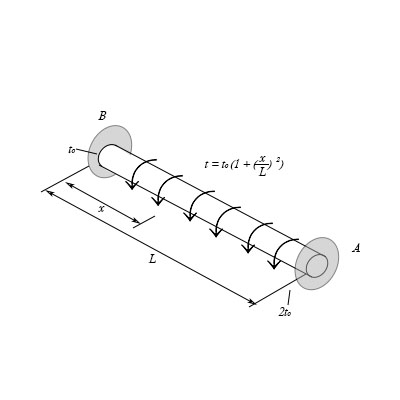# The shaft of radius c is subjected to a disturbed torque t, measured as torque\length of shaft....

## Question:

The shaft of radius {eq}c {/eq} is subjected to a disturbed torque {eq}t {/eq}, measured as torque\length of shaft.

Part A

Determine the reaction at the fixed support {eq}A {/eq}.

Express your answer in terms of the variables {eq}t_o {/eq} and {eq}L {/eq}.

Part B

Determine the reaction at the fixed support {eq}B {/eq}.

Express your answer in terms of the variables {eq}t_o {/eq} and {eq}L {/eq}.## Torque

It is defined as the rotation occurred due to applied force at a certain distance. Mathematically torque is defined as the product of force and distance with Sin angle. Maximum torque is occurred when angle is {eq}90^\circ {/eq}. It is measured in Newton meter.

## Answer and Explanation:

Given data:

• The radius of shaft is: {eq}c {/eq}
• The length of shaft is: {eq}L {/eq}
• The distributed torque on shaft is: {eq}t = {t_0}\left( {1 + {{\left( {\dfrac{x}{L}} \right)}^2}} \right) {/eq}

The expression for the torque at distance x is,

{eq}{T_x} = \int\limits_0^x {tdx} {/eq}

Substitute the given values in above expression.

{eq}{T_x} = \int\limits_0^x {{t_0}\left( {1 + {{\left( {\dfrac{x}{L}} \right)}^2}} \right)dx} {/eq}

Integrate the above equation.

{eq}\begin{align*} {T_x} &= \left[ {{t_0}\left( {x + \dfrac{{{x^3}}}{{3{L^2}}}} \right)} \right]_0^x\\ &= {t_0}\left( {x + \dfrac{{{x^3}}}{{3{L^2}}}} \right) \end{align*} {/eq}

The expression for the rotation due to applied torque is,

{eq}\begin{align*} \theta &= \dfrac{{\int\limits_0^L {{t_0}\left( {x + \dfrac{{{x^3}}}{{3{L^2}}}} \right)dx} }}{{JG}}\\ &= \dfrac{{{t_0}\left( {\dfrac{{{x^2}}}{2} + \dfrac{{{x^4}}}{{12{L^2}}}} \right)_0^L}}{{JG}}\\ &= \dfrac{{{t_0}\left( {\dfrac{{{L^2}}}{2} + \dfrac{{{L^2}}}{{12}}} \right)}}{{JG}}\\ &= \dfrac{{7{t_0}{L^2}}}{{12JG}} \end{align*} {/eq}

Here, {eq}G {/eq} is modulus of rigidity and {eq}J {/eq} is polar moment of inertia.

Both the end of the shaft is fixed so angle of rotation should be zero then the applied torque is equal to torque at point B.

{eq}\theta = {\theta _B} {/eq}

Substitute the given values in above expression.

{eq}\begin{align*} \dfrac{{7{t_0}{L^2}}}{{12JG}} &= \dfrac{{{T_B}L}}{{JG}}\\ {T_B}& = \dfrac{{7{t_0}L}}{{12}} \end{align*} {/eq}

The expression for the net torque is,

{eq}{T_A} + {T_B} = \int\limits_0^L {{T_x}} dx {/eq}

Substitute the given values in above expression.

{eq}\begin{align*} {T_A} + \dfrac{{7{t_0}L}}{{12}} &= \int\limits_0^L {{t_0}\left( {1 + {{\left( {\dfrac{x}{L}} \right)}^2}} \right)} dx\\ {T_A} + \dfrac{{7{t_0}L}}{{12}} &= \left[ {{t_0}\left( {x + {{\left( {\dfrac{{{x^3}}}{{3{L^2}}}} \right)}^2}} \right)} \right]_0^L\\ {T_A} + \dfrac{{7{t_0}L}}{{12}} &= {t_0}\left( {L + {{\left( {\dfrac{L}{3}} \right)}^2}} \right)\\ {T_A} &= \dfrac{{3{t_0}L}}{4} \end{align*} {/eq}

(A)

Thus, reaction at A is {eq}{T_A} = \dfrac{{3{t_0}L}}{4}. {/eq}

(B)

Thus, the reaction at B is {eq}{T_B} = \dfrac{{7{t_0}L}}{{12}}. {/eq}

#### Learn more about this topic:Torque: Concept, Equation & Example

from UExcel Physics: Study Guide & Test Prep

Chapter 7 / Lesson 4
19K